• 哈希表线性探测法和二次探测法详细代码
千次阅读
2018-07-28 16:58:14
#include <stdio.h>
#include <stdlib.h>
#include <windows.h>
#include <assert.h>

typedef int Key;

typedef int(*HashFunc)(Key, int);
//定义每个位置的状态信息
typedef enum {
EXISTED,
DELETED,
EMPTY
}	State;

//哈希表中每个元素不仅包含关键字，还有其状态信息
typedef struct Element{
Key key;
State state;
}	Element;

//哈希表，包括元素类型，有效数据大小，容量和哈希函数
typedef struct HashTable {
Element *arr;
int size;
int capacity;
HashFunc hashfunc;
}	HashTable;

//初始化，初始化表的大小，哈希函数，元素关键字和状态
void HashInit(HashTable *Hash, int capacity, HashFunc hashfunc)
{
assert(Hash);
Hash->arr = (Element *)malloc(sizeof(Element)*capacity);
assert(Hash->arr);
int index;
for (index = 0; index < capacity; index++) {
Hash->arr[index].key = 0;
Hash->arr[index].state = EMPTY;
}

Hash->size = 0;
Hash->capacity = capacity;
Hash->hashfunc = hashfunc;
}

//查找，传入想要查找的关键字，通过hashfunc计算出可能的下标
//首先数据在表中插入的时候先判断当前位置是否为空，如果不为空就接着往后直到找到空位置
//所以在查找的时候只需要查找从当前位置到往后第一个状态为空的位置，如果遇到空了还没找到就表示不存在
//需要注意查找到的第一条件是当前位置状态不为EMPTY，第二条件是状态为EXISTED而不是DELETED
//其次才是比较关键字是否相等
//查找的时候hashfunc开始计算的下标可能不是表的开头位置，所以需要将下标循环起来，以确保能查找到每个位置
//但是这样的话可能出项死循环，只需要判断查找次数是否大于capacity就行了
int Search(HashTable *Hash, Key key)
{
int index = Hash->hashfunc(key, Hash->capacity);
int iCount = 0;
while (Hash->arr[index].state != EMPTY) {
if (Hash->arr[index].key == key && Hash->arr[index].state == EXISTED) {
return 1;
}

//缺点 容易出现死循环
index = (index + 1) % Hash->capacity;
iCount++;
if (iCount >= Hash->capacity) {
return -1;
}
}
return -1;
}

//由于负载因子的存在，在表中有效数据占总容量的比例不得大于0.7
//所以在插入之前需要判断是否要扩容
void ExpandIfNeed(HashTable *Hash);

//通过关键字计算出下标，
int Insert(HashTable *Hash, Key key)
{
ExpandIfNeed(Hash);

int iCount = 1;
int index = Hash->hashfunc(key, Hash->capacity);

//while循环的作用是找到表中状态不为EXISTED的位置
while (Hash->arr[index].state == EXISTED) {
//确保已存在的key不是处于假删除状态
if (Hash->arr[index].key == key && Hash->arr[index].state == EXISTED) {
return -1;
}

//二次探测法
index = (index + iCount*iCount) % Hash->capacity;
iCount++;

}

Hash->arr[index].key = key;
Hash->arr[index].state = EXISTED;

return 1;
}

//扩容操作
//如果负载因子超标，则执行次操作
//初始化一个容量为之前二倍的新标
//将原来的元素重新按规则而不是无脑插入到新表中
//完成之后释放旧表，拿到新表
void ExpandIfNeed(HashTable *Hash)
{
assert(Hash);
if (Hash->size * 10 / Hash->capacity < 7) {
return;
}

HashTable newHash;
HashInit(&newHash, Hash->capacity * 2, Hash->hashfunc);

int i = 0;
for (i = 0; i < newHash.capacity; i++) {
if (Hash->arr[i].state == EXISTED) {
Insert(&newHash, Hash->arr[i].key);
}
}

free(Hash->arr);
Hash->arr = newHash.arr;
Hash->capacity = newHash.capacity;
}

//删除------假删除
//只所以要假删除是因为在表中数据不是连续排列的
//而查找的操作可能要对表中的位置挨个进行访问，如果遇到EMPTY的结点就会退出
int Remove(HashTable *Hash, Key key)
{
assert(Hash);

int index = Hash->hashfunc(key, Hash->capacity);
while (Hash->arr[index].state != EMPTY) {
if (Hash->arr[index].key == key && Hash->arr[index].state == EXISTED) {
Hash->arr[index].state = DELETED;
Hash->size--;
return 1;
}

index = (index + 1) % Hash->capacity;
}
return -1;
}

void Destory(HashTable *Hash)
{
free(Hash->arr);
Hash->arr = NULL;
}

//哈希函数，除留余数法
//还有直接定制法， 平方取中法，折叠法，随机数法，数学分析法
//哈希函数越巧妙，哈希碰撞的几率就越低，但是不可避免
int chuliuyushufa(Key key, int capacity) {
return key % capacity;
}

void HashPrint(HashTable *Hash)
{
assert(Hash);

int index = 0;
for (index = 0; index < Hash->capacity; index++) {
printf("key : %-2d	index : %-2d\n", Hash->arr[index].key, index);
}
}

int main()
{
HashTable Hash;
HashInit(&Hash, 13, chuliuyushufa);
Insert(&Hash, 3);
Insert(&Hash, 7);
Insert(&Hash, 19);
Insert(&Hash, 25);
Insert(&Hash, 26);
Insert(&Hash, 6);
Insert(&Hash, 12);
Insert(&Hash, 39);
Insert(&Hash, 41);
Insert(&Hash, 32);
HashPrint(&Hash);

//走到这一步会触发扩容
Insert(&Hash, 45);
HashPrint(&Hash);

system("pause");
return 0;
}


更多相关内容
• 构造哈希表（散列表）以及二次探测法 今天做笔试题时，遇到一道构造哈希表的题，hash函数是 k%11 ，然后一个数组记不清了，然后就是问二次探测法进行，问下面那个是正确，懵逼啊，没做过，不知道，乱选直接下...

今天做笔试题时，遇到一道构造哈希表的题，hash函数是 k%11 ，然后一个数组记不清了，然后就是问二次探测法进行，问下面那个是正确，懵逼啊，没做过，不知道，乱选直接下一题，于是有这个博客，赶紧学习一波。

网上查询了一下。

### 构造哈希表的几种方法

常用方法是直接定址法除留余数法

• 直接定址法（取关键字的某个线性函数为哈希地址）

类似于这样的式子

f(key) = a × key + b

• 除留余数法（取关键值被某个不大于散列表长m的数p除后的所得的余数为散列地址）

对于散列表长为m的散列函数公式为：

f( key ) = key mod p ( p ≤ m )

mod是取模（求余数）的意思。事实上，这方法不仅可以对关键字直接取模，也可在折叠、平方取中后再取模。

• 平方取中法

• 折叠法

• 随机数法

• 数学分析法

### 哈希冲突（碰撞）以及处理

哈希冲突：既然有哈希函数Hash(key)，在有限的空间里，肯定会产生相同的的值（哈希地址），我们称这种情况为哈希冲突（碰撞）。任意的散列函数都不能避免产生冲突。

#### 1. 开发定址法

所谓的开放定址法就是一旦发生了冲突，就去寻找下一个空的散列地址，只要散列表足够大，空的散列地址总能找到，并将记录存入。

• 线性探测法

fi(key) = (f(key)+di) MOD m (di=1,2,3,......,m-1)

用开放定址法解决冲突的做法是：当冲突发生时，使用某种探测技术在散列表中形成一个探测序列。沿此序列逐个单元地查找，直到找到给定的关键字，或者碰到一个开放的地址（即该地址单元为空）为止（若要插入，在探查到开放的地址，则可将待插入的新结点存人该地址单元）。查找时探测到开放的地址则表明表中无待查的关键字，即查找失败。

ep:我们的关键字集合为{12,67,56,16,25,37,22,29,15,47,48,34},表长为12。 我们用散列函数f(key) = key mod 12。

当计算前S个数{12,67,56,16,25}时，都是没有冲突的散列地址，直接存入：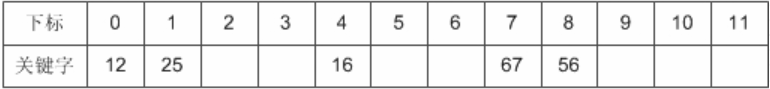计算key = 37时，发现f(37) = 1，此时就与25所在的位置冲突。

于是我们应用上面的公式f(37) = (f(37)+1) mod 12 = 2。于是将37存入为2的下标位置。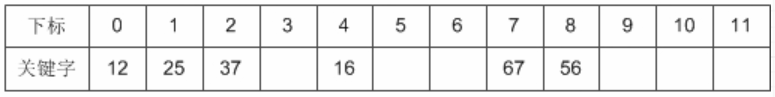接下来22,29,15,47都没有冲突，正常的存入：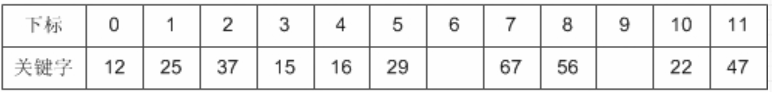到了 key=48，我们计算得到f(48) = 0，与12所在的0位置冲突了，不要紧，我们f(48) = (f(48)+1) mod 12 = 1，此时又与25所在的位置冲突。于是f(48) = (f(48)+2) mod 12=2，还是冲突……一直到 f(48) = (f(48)+6) mod 12 = 6时，存入该位置：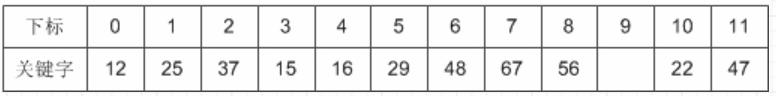我们把这种解决冲突的开放定址法称为线性探测法。

• 二次探测法

考虑深一步，如果发生这样的情况，当最后一个key=34，f(key)=10,与22所在的位置冲突，可是22后面没有空位置了，反而它的前面有一个空位置，尽管可以 不断地求余数后得到结果，但效率很差。

因此我们可以改进di = 12, -12, 22, -22,……, q2, -q2 (q <= m/2),这样就等于是可以双向寻找到可能的空位置。

对于34来说，我 们取di即可找到空位置了。另外增加平方运算的目的是为了不让关键字都聚集在 某一块区域。我们称这种方法为二次探测法。

f(key) = (f(key)+di) MOD m (di = 1^2, -1^2, 2^2, -2^2,……, q^2, -q^2, q <= m/2)

注：1^2 表示是 1的平方。

### 2. 链地址法

前面我们谈到了散列冲突处理的开放定址法，它的思路就是一旦发生了冲突，就去寻找下一个空的散列地址。那么，有冲突就非要换地方呢，我们直接就在原地处理行不行呢？

答案是可以的，就是链地址法，就好比Java里的HashMap的数据结构一样。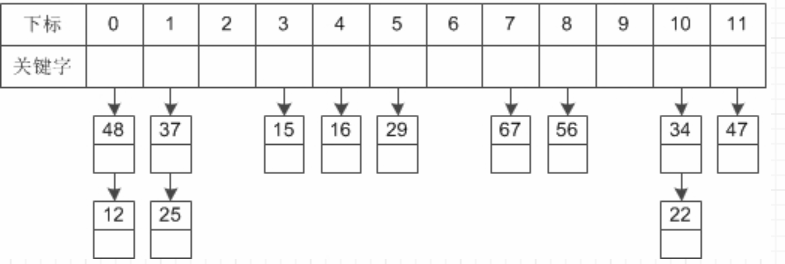关于HashMap源码分析 ——> https://blog.csdn.net/Stu_zkl/article/details/82714325
好了，就写到这了。

展开全文Java
• 　（2）从键盘读入待查找的权重数值，以除留余数为哈希函数，二次探测再散列解决冲突建立哈希表，基于哈希算法从数组中查找相应的记录，计算相应的查找时间，并在屏幕上输出显示。(提示:当前计算机时间 函数 C\...
• ## 构造哈希表之二次探测法

万次阅读 多人点赞 2016-06-08 01:19:00
HashTable-散列表/哈希表 是根据关键字（key）而直接访问在内存存储位置的数据结构。 它通过一个关键值的函数将所需的数据映射到表中的位置来访问数据，这个映射函数叫做散列（哈希）函数，存放记录的数组叫做散列表...

HashTable-散列表/哈希表

是根据关键字（key）而直接访问在内存存储位置的数据结构。

它通过一个关键值的函数将所需的数据映射到表中的位置来访问数据，这个映射函数叫做散列（哈希）函数，存放记录的数组叫做散列表。

构造哈希表的几种方法
1.直接定址法（取关键字的某个线性函数为哈希地址）
2.除留余数法（取关键值被某个不大于散列表长m的数p除后的所得的余数为散列地址）
3.平方取中法
4.折叠法
5.随机数法
6.数学分析法
常用方法是 直接定址法除留余数法

哈希冲突/哈希碰撞
不同的Key值经过哈希函数Hash(Key)处理以后可能产生相同的值哈希地址，我们称这种情况为哈希冲突。任意的散列函数都不能避免产生冲突。

处理哈希碰撞的方法
若key1,key2,key3产生哈希冲突(key1,key2,key3值不相同，映射的哈希地址同为key)，用以下方法确定它们的地址

1.闭散列法
1）线性探测
若当前key与原来key产生相同的哈希地址，则当前key存在该地址之后没有存任何元素的地址中
key1：hash(key)+0
key2：hash(key)+1
key3：hash(key)+2
例如：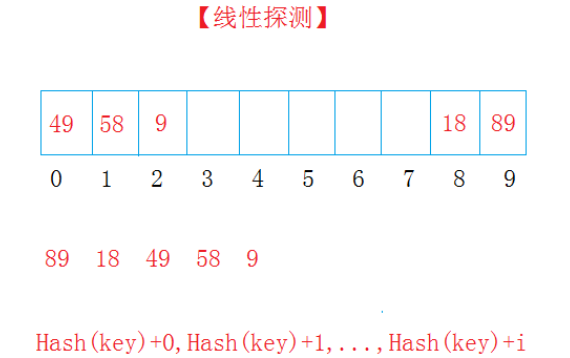2）二次探测
若当前key与原来key产生相同的哈希地址，则当前key存在该地址后偏移量为（1,2,3...）的二次方地址处
key1：hash(key)+0
key2：hash(key)+1^2
key3：hash(key)+2^2
例如：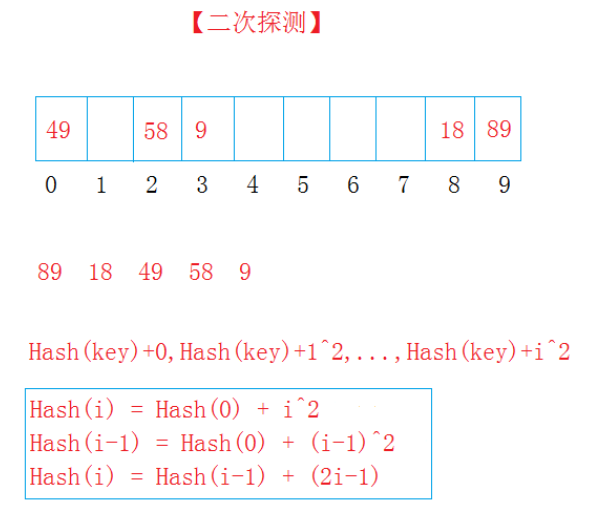2.开链法（哈希桶）
哈希表中保存包含每个key值的节点，每个节点有一个_next的指针，指向产生哈希冲突的key的节点
例如：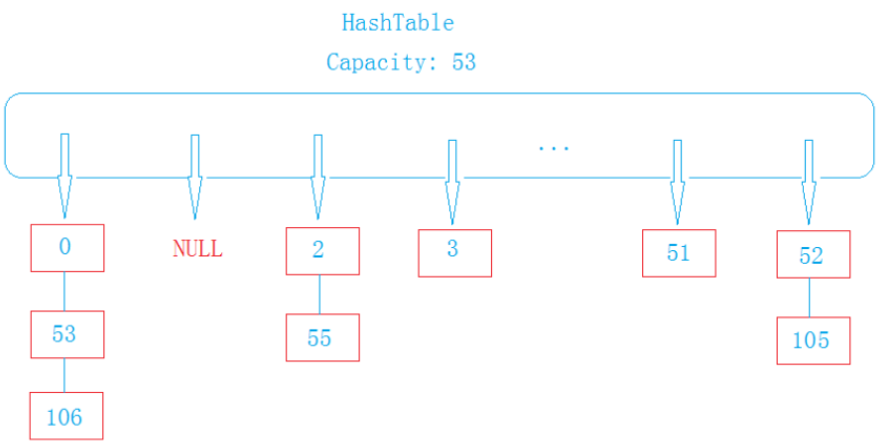具体实现方式请看下一篇博客，博客链接： http://blog.csdn.net/xyzbaihaiping/article/details/51610944

构建哈希表（二次探测法）
支持key值为字符串
<pre name="code" class="cpp">//HashTable.h
#pragma once
#include<iostream>
#include <string>
using namespace std;
enum State
{
EMPTY,//空
EXITS,//存在
DELETE//已删除
};

template<class K, class V>
struct HashTableNode
{
K _key;
V _value;
};

template<class K>
struct _HashFunc
{
size_t operator()(const K& key,const size_t& capacity)//哈希函数，仿函数
{
return key / capacity;
}

};
template<>
struct _HashFunc<string>//模板特化
{
private:
unsigned int _BKDRHash(const char *str)//key为字符串时哈希函数
{
unsigned int seed = 131; // 31 131 1313 13131 131313 etc..
unsigned int hash = 0;

while (*str)
{
hash = hash * seed + (*str++);
}
return (hash & 0x7FFFFFFF);
}
public:
size_t operator()(const string& key,const size_t& capacity)//仿函数
{

return _BKDRHash(key.c_str()) % capacity;
}

};
template<class K, class V,class HashFunc=_HashFunc<K>>
class HashTable
{

typedef HashTableNode<K, V> Node;
public:
HashTable(size_t capacity = 10)
:_tables(new Node[capacity])
, _states(new State[capacity])
, _size(0)
, _capacity(capacity)
{}
~HashTable()
{
if (_tables != NULL)
{
delete[] _tables;
delete[] _states;
}

}
HashTable(const HashTable<K, V>& ht)
{
HashTable<K, V> tmp(ht._capacity);
for (size_t i = 0; i < ht._capacity; i++)
{
tmp.Insert(ht._tables[i]._key, ht._tables[i]._value);
}
this->Swap(tmp);
}
HashTable& operator=(HashTable<K, V> ht)
{
this->Swap();
return *this;
}
bool Insert(const K& key, const V& value)
{
_CheckCapacity();
size_t index = HashFunc()(key, _capacity);
size_t i = 1;
while (_states[index] == EXITS)//二次探测
{
if (_tables[index]._key == key)
{
return false;
}
index = index + 2 * i - 1;
index %= _capacity;
++i;
}
_tables[index]._key = key;
_tables[index]._value = value;
_states[index] = EXITS;
++_size;
return true;
}

bool Find(const K& key)
{
size_t index = HashFunc()(key, _capacity);
size_t start = index;
size_t i = 1;
while (_states[index] != EMPTY)//根据二次探测法查找
{
if (_tables[index]._key == key)
{
if (_states[index] != DELETE)
return true;
else
return false;
}
index = index + 2 * i - 1;
index %= _capacity;
if (start == index)
return false;
}
return false;
}
bool Remove(const K& key)
{
size_t index = HashFunc()(key, _capacity);
size_t start = index;
size_t i = 1;
while (_states[index] != EMPTY)//根据二次探测法删除
{
if (_tables[index]._key == key)
{
if (_states[index] != DELETE)
{
_states[index] = DELETE;
_size--;
return true;
}
else
return false;
}
index = index + 2 * i - 1;
index %= _capacity;
if (start == index)
return false;
}
return false;

}
void Print()
{
for (size_t i = 0; i < _capacity; i++)
{
//printf("%d-[%s:%s] \n", _states[i], _tables[i]._key, _tables[i]._value);
cout << _states[i] << " " << _tables[i]._key << " " << _tables[i]._value<<endl;
}
}
private:
void Swap(HashTable<K, V>& tmp)
{
swap(_tables, tmp._tables);
swap(_states, tmp._states);
swap(_size, tmp._size);
swap(_capacity, tmp._capacity);
}
void _CheckCapacity()//增容
{
if (_size * 10 / _capacity == 6)
{
HashTable<K, V> tmp(_capacity * 2);
for (size_t i = 0; i < _capacity; i++)
{
if (_states[i] == EXITS)
tmp.Insert(_tables[i]._key, _tables[i]._value);
}
this->Swap(tmp);
}
}

private:
Node* _tables;//哈希表
State* _states;//状态表
size_t _size;
size_t _capacity;
};


</pre><pre code_snippet_id="1711228" snippet_file_name="blog_20160608_3_3809584" name="code" class="cpp">//test.cpp
#include<iostream>
#include "HashTable.h"
void testInt()
{
HashTable<int, int> table(10);
table.Insert(89, 89);
table.Insert(18, 18);
table.Insert(49, 49);
table.Insert(58, 58);
table.Insert(9, 9);
//table.Insert(45, 45);
//table.Insert(2, 2);
table.Print();
HashTable<int, int> table1(table);
table1.Print();
bool ret = table.Find(9);
cout << endl << ret << endl;
table.Remove(9);
table.Print();

}
void TestString()
{
HashTable<string, string> table(10);
table.Insert("dict", "字典");
table.Insert("hash", "哈希");
table.Insert("function", "函数");
table.Insert("abcd", "函数");
table.Insert("dcba", "函数");

table.Print();
bool ret = table.Find("function");
cout << endl << ret << endl;
table.Remove("hash");
table.Print();
}
int main()
{
//testInt();
TestString();
getchar();
return 0;
}

测试结果：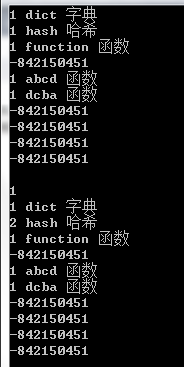展开全文闭散列法
•代码
• 选题是哈希表的相关简单应用，假设模拟了某系统前端登录采用8个英文字母组成的密码，为防止密码明文被窃取，后台系统验证模块采用长度为m=10的哈希表进行加密、存储和验证，采用除p留余法和二次探测法将密码明文中的...

### 前言

选题是哈希表的相关简单应用，假设模拟了某系统前端登录采用8个英文字母组成的密码，为防止密码明文被窃取，后台系统验证模块采用长度为m=10的哈希表进行加密、存储和验证，采用除p留余法和二次探测法将密码明文中的各个英文字母按输入顺序散列到哈希表中，其余空白单位默认为0，最终得到10字节的不可逆杂文作为加密结果。

选题的设计要求，输入的明文密码中8为英文字母不可以出现相同的英文字母，模拟登录输入密码，代入哈希算法与已存储的不可逆杂文比对，实现密码校验，显示验证是否成功。针对后台加密存储的不可逆杂文进行模拟破解，采用哈希碰撞的方法，即任何数据，哪怕只有多或者少了空格都有可能对哈希值进行改变，此即为哈希的碰撞特性，通过此特性，检测出所有可能验证通过的密码明文。

### 代码

以下展示主代码部分，详细内容可以私信

void Insert_hashNum(hashTable1 t,hashTable h,int m,int data,int p){//将字符插入哈希表
int index,i=0;
int q=0,w=0;
index=data%p;
if(h[index].key==NULL){
h[index].key=data;
h[index].count=1;
//	printf("index=%d h[].key=%d h[index].count=%d\n",index,h[index].key,h[index].count);
}
else{
i=1,q=0;
while(h[index].key!=NULL){
index=data;
//	printf("q=%d   t[]=%d ",q,t[q].ps);
index=(data+t[q].ps)%m;//t[q].ps中存储了等比数列1，-1，4，-4，9，-9...
//	printf("  index=%d\n",index);
i++;
q++;
}
h[index].key=data;
h[index].count=i;
//	printf("index=%d h[].key=%d h[index].count=%d\n",index,h[index].key,h[index].count);
}
}

展开全文• //偶数冲突向后查找 NewPos = CurrentPos - ( CrashNum / 2 ) * ( CrashNum / 2 ) ; while ( NewPos < 0 ) //等于也不行 { NewPos = NewPos + h - > TableSize...
• 假设哈希表长度为n,哈希函数为Hash(key)=key%n, key为关键码。 当Hash(key)相等时，则使用Hash(key） = (Hash(key) + d) % n,d为：1^2、-(1 ^2)、2 ^2、-(2 ^2)、3 ^2、-(3 ^2)… 例子： 设哈希表长为11，哈希函数为...
• HashTable叫哈希表或者散列表。 它通过一个关键值的函数将所需的数据直接映射到表中的位置来访问数据，这个映射函数叫散列函数（哈希函数），存放记录的数组叫散列表（哈希表）。比如： 给定一字符串...hashtable 散列函数 数据结构
• 哈希表-线性探测法理论 线性探测法的理论我们在上一篇博客已经阐述了。 现在我们来看看线性探测法的增删查的代码思想： 哈希表的增加元素： 注意：往后遍历寻找空闲位置的时候，要注意是环形遍历哦！不然访问数组...算法
•数据结构
• ## 二次探测法

万次阅读 多人点赞 2020-05-04 16:02:36
存在关键码{43,7,29,22,16,92,44,8,19}，采用二次探测法处理冲突，建立的hash为（ ） 二次探测法：采用开放定址法处理冲突中的二次探测再散列（也即是题目中的二元探测法）,则哈希函数变为Hash(key） = (Hash(key)...
• 哈希表又称散列表。 哈希表存储的基本思想是：以数据表中的每个记录的关键字 k为自变量，通过一种函数H(k)计算出函数值。把这个值解释为一块连续存储空间（即数组空间）的单元地址（即下标），将该记录存储到这个...
• 散列表（Hash table，也叫哈希表），是根据关键码值(Key value)而直接进行访问的数据结构。也就是说，它通过把关键码值映射到表中一个位置来访问记录，以加快查找的速度。这个映射函数叫做散列函数，存放记录的数组...线性探测
• 输入表长（大于、等于11），输入关键字集合，用二次探测再散列构建哈希表，并查找给定关键字。 输入 测试次数t 每组测试数据格式如下： 哈希表长m、关键字个数n n个关键字 查找次数k k个待查关键字 输出 对每组测试...数据结构 算法 hash
• #include<iostream> #include<time.h> #include<string> #include<math.h>...#define m 15//哈希表的表长 ...//HT是哈希表，HC是统计每个元素的插入时候的查找次数。并且初始化。 int H
• 本文为大家分享了C语言基于哈希表实现通讯录的具体代码，供大家参考，具体内容如下 1．需求分析 本演示程序用C语言编写，完成哈希表的生成，电话号码的插入、以及查找等功能。 　(1)按提示输入相应的联系人的相关...
• 哈希表：线性探测法和链地址法求查找成功与不成功的平均查找
• 在代码中实现了哈希表中任意类型都可以存放，即哈希函数要可扩展以及哈希表动态增容的功能。 贴上代码： #include #include using namespace std; template//特化 class _HashFun { public: size_t operator...数据结构
• 什么是哈希表 哈希表用的是 数组支持按照下标随机访问数据的特性 ，所以哈希表其实就是数组的一种扩展，由数组演化而来。 可以说，如果没有数组，就没有散列表。 哈希表存储的是由键（key）和值（value）组成的数据...散列表 数据结构
• 假设数组hashTable的长度是mSize，用平方探测法插入数字num，每次的位置pos = (num + i * i) % mSize，i的范围是[0, mSize)。 bool flag = false; //未插入 for(int i = 0; i < mSize; i++){ int pos = (num + i...
• 哈希表是一种搜索结构，当数据量大时，哈希搜索的效率高，平均时间复杂度O(1)。 【哈希查找】： （1）在插入时，根据待插入元素的关键码，以此函数计算出该元素的存储位置并按此位置进行存放。 （2）在搜索时，对...
• 文章目录 题目分析 题目链接 题目分析 来源:acwing 分析： 本题建立hash表是利用只具有正增量的二次探测法来解决冲突， 索引 = 数 % 哈希表的大小 如果映射到同一个索引idx，hash表的长度是s，需要解决冲突：先看 ...
• 理想的搜索方法是可以不经过任何比较，一直接从中得到要搜索的元素。如果构造一种存储结构，使元素的存储位置与它的关键码之间建立一个确定的对应函数关系Hash()，那么每个元素关键码与结构中的一个唯一的存储.....
• 一、哈希表 1、概念 哈希表(Hash Table)也叫散列表，是根据关键码值（Key Value）而直接进行访问的数据结构。它通过把关键码值映射到哈希表中的一个位置来访问记录，以加快查找的速度。这个映射函数就做散列函数，...数据结构
• 分别画出用线性探测法和拉链法解决冲突时构造的哈希表，并求出在等概率情况下，这两种方法的查找成功和查找不成功的平均查找长度。 2.链地址法：用链地址发解决冲突的方法时：把所有关键字为同义词的记录...
• 1078 Hashing (25 分) 题目传送门：1078 Hashing (25 分) ...在哈希时如果冲突，则二次探测再散列。刚开始没注意这一句:Quadratic probing (with positive increments only) is used to solve the...
• 问题描述从空表开始，将输入元素按照输入顺序逐个插入一个哈希表，以生成哈希表...=m)，用二次探测再散列处理冲突，即探测序列为Hi=(Hash(key)+di) mod m，其中增量序列为di = 12, -12, 22, -22,32,-32…, k2, -k2......